# Community

Blog Events Webinars Tutorials Forum
×
Community Blog Intuitive Comparison of Four NLP Models - Neural Network, RNN, CNN, and LSTM

# Intuitive Comparison of Four NLP Models - Neural Network, RNN, CNN, and LSTM

By Zijin, from F(x) Team

The differences between each model used in natural language processing (NLP) are difficult to grasp sometimes because they have similarities. Also, the use of new models is usually envisaged to overcome the shortcomings of previous models. Therefore, this article will delve into the nature of each model and introduce its advantages and disadvantages.

After having a basic understanding of how models work, we will try the NLP classification (sentiment analysis) exercise in the second part of this article and see how each model is stacked. Hopefully, you will have a deep understanding of the most common NLP models by the end of this article.

The content of this article is mainly based on the CS224N course at Stanford University. The course is highly recommended to everyone interested in NLP. Each section will provide additional links to relevant papers and articles for you to delve into the topic.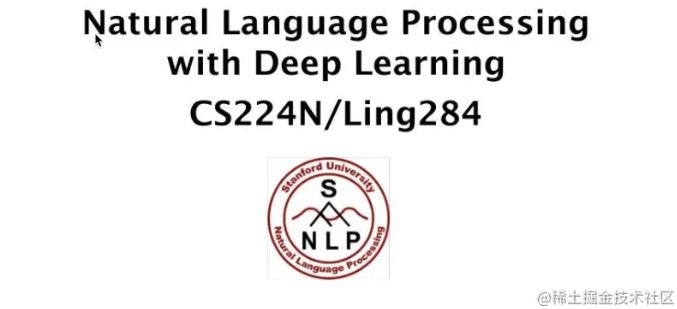## Neural Network

Expanding Reading: Michael Nelson's Neural Networks and Deep Learning

### How Does It Work?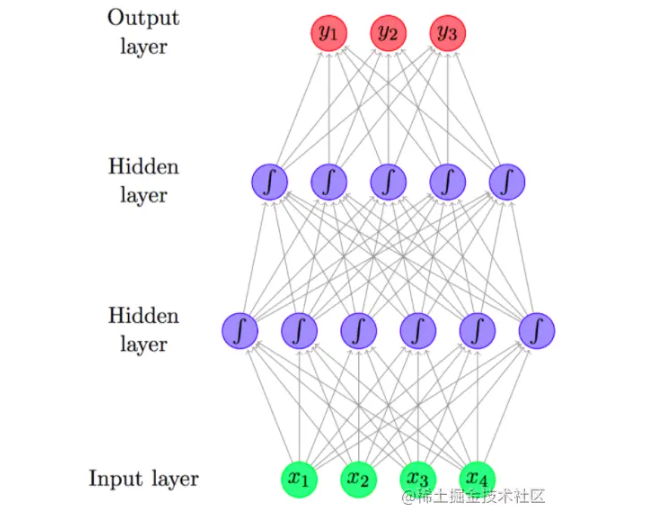(Feed-forward neural networks, source: A Primer on Neural Network Models for Natural Language Processing)

1. With a sequence of words as input, the number of words is fixed, which is called a fixed window.
2. For each word, look for word embedding to convert the word into a vector and then connect the word vectors to get a matrix.
3. The hidden layer is obtained by passing the connective matrix through a linear layer and a nonlinearity function.
4. The output distribution probability is obtained by placing the hidden layer in another linear layer and activation function, such as softmax or tanh. The model can learn the nonlinear interaction between input word factors by having an additional hidden layer.

### The Problem of Neural Networks

1. The fixed window may be too small. For example, consider the sentence above. If we enter five words in the fixed window, we will get "my day started off terrific" and ignore the context in the rest of the sentence.
2. Expanding the fixed window complicates the model. If we expand the model, the weight matrix used to calculate the linear layer will expand. If we perform a lengthy review operation, the window will never be large enough to accommodate all the context.
3. Since the weight of each word is independent, every time one of the weight matrix columns is adjusted to one word, the other columns will not be adjusted accordingly, which will make the model inefficient. This is a problem since the words in the sentence should have something in common. Learning each word individually will not take advantage of this feature.

## Recurrent Neural Network (RNN)

Researchers conceived RNN to understand the sequential characteristics of human language and improve traditional neural networks. The basic principle of RNN is to browse each word vector from left to right and keep the data of each word so that when we reach the last word, we can still get information about the previous word.

### How Does It Work?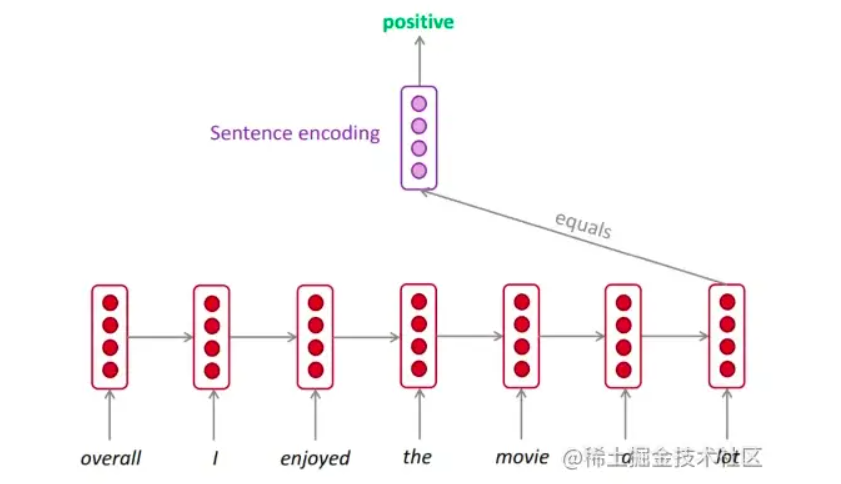(RNN practice in sentiment analysis, source: Stanford CS224N lecture slide)

1. Select a sequence of words (sentences) as input
2. Find the word embedding to convert the word to a vector. Unlike neural networks, RNN does not connect all word vectors into one matrix since the purpose of RNN is to absorb information from each word vector separately to obtain sequential information.
3. For each step (each word vector), we use the current hidden state (multiplied by the weight matrix) and the information in the new word vector to calculate the current hidden state. When the model reaches the last word of the text, we will get a hidden state that contains information from the previous hidden state (and the previous word).
4. In terms of the problem we are solving, there are several ways to calculate the output. For example, if we are studying a language model (find the direct word after a sentence), we can place the last hidden state through another linear layer and activation function. As such, it makes sense to use the last hidden state since the next word may be the most relevant to the previous word. If we are doing sentiment analysis, we can use the last hidden state, but a more effective method is to calculate the output state for all the previous hidden states and obtain the average of these output states. The basic principle of using the output state of all previously hidden states is that the overall mood may be implied anywhere in the text (not just from the last word).

1. It can handle any length of the input, which solves the problem of the fixed window size of the neural network.
2. Applying the same weight matrix to each input is a problem faced by neural networks. Having the same weight matrix makes it more effective to use RNN to optimize the weight matrix.
3. The model size remains constant for any length of the input.
4. In theory, the RNN would still consider many words at the front of a sentence, which would provide the model with context for the entire text. The context will be important in our sentiment analysis exercises for film reviews. Reviewers may often include positive emotions (the special effects are great) and negative sentences (the performance is terrible) in a single review. Therefore, this model needs to understand the background of the entire review.

### Problems of RNN

1. Since each implied state cannot be calculated before the previous implied state is calculated, the RNN calculation is slow. Unlike neural networks, RNNs cannot be calculated in parallel. Training RNN models consume a lot of resources.
2. Vanishing Gradient Problem: Vanishing gradient problem refers to the phenomenon that the earlier hidden state has less influence on the final hidden state, thus weakening the influence of the earlier word on the whole context. This means the information from the older hidden state will continue to pass to the newer hidden state, but the information will become smaller in each new hidden state. For example, if the first hidden state=  and the weight matrix= [0.1], the effect of the first hidden state on the second hidden state will be [first hidden state x weight matrix] = [0.1]. In the third hidden state, the effect of the first hidden state on the third hidden state is [0.1 x 0.1] = [0.01]. This suggests the effect of the older hidden state will become smaller until the effect is not obvious. The vanishing gradient problem can be deduced mathematically by calculating the gradient of the loss concerning any hidden state using the chain rule. If you are interested, Denny Britz's article will provide a detailed explanation.

Researchers have developed many variants of RNN to solve these problems. This article discusses LSTM and briefly introduces Bidirectional RNN. You can also study more examples by yourself, such as multi-layer RNN and GRU.

## Long and Short-Term Memory (LSTM)

LSTM is a response solution to the problem of vanishing gradients in RNN. Do you remember how the RNN tried to preserve the information in the previous word? The principle behind LSTM is that the machine will learn the importance of previous words so we will not lose information from earlier hidden states. Colah's illustrations are excellent as a detailed guide to LSTM.

### How Does It Work?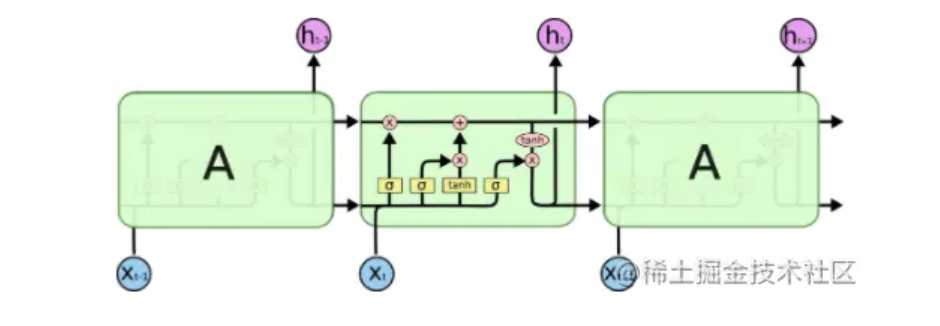1. Select a sequence of words (sentences) as input
2. Find every word embedding to convert the word to a vector
3. For each step (word embedding), we choose the information to forget from the previous state (for example, if the previous sentence is in the past tense and the current sentence is in the current tense, we forget the fact that the previous sentence is in the past tense because it has nothing to do with the current sentence) and what information to remember from the current state. All this information is stored in a new state called the cell state, which serves to carry past information since it should be remembered.
4. Each step has a hidden state, which obtains information from the cell state to determine what to output for each word.

LSTM can preserve the information at the front of the sentence, so it provides the gradient vanishing problem of RNN.

### Problem

1. Like RNN, LSTM fails to solve the parallelization problem of RNN, as each hidden state and cell state must be calculated before the next hidden state and cell state are calculated. This also means LSTM takes longer to train and requires more memory.
2. So far, both RNN and LSTM have described one direction: from left to right. This means we lack the context of words we have not seen yet (mainly the context below) for tasks such as Machine Translation. This drives the derivation of another variant of RNN and LSTM, which we call bidirectional RNN or bidirectional LSTM. Since it is useful in understanding the context, bidirectional LSTM is often seen in papers and applications.

## Add an Attention Layer to LSTM

This part is based on the paper entitled Attention is All You Need (from Google) and the paper entitled Hierarchical Attention Networks for Document Classification (jointly produced by CMU and Microsoft).

First, we use a paragraph from Attention is All You Need to explain the concept of attention:

Self-attention, sometimes called intra-attention, is an attention mechanism related to different positions of a single sequence. The purpose is to calculate the representation of the sequence.

Let's suppose we want to translate "the table is red" into French. Note: We give each word in the original sentence an attention vector. For example, "the" will have an attention vector, "table" will have an attention vector, etc. In the attention vector of each word, assuming the attention vector of the, we have the element of each word in the sentence. The nth element in the attention vector represents the nth word and the word the. For example, if the attention vector of the is [0.6, 0.3, 0.07, 0.03], the is the most related word (the original word is most related to itself), while red is the least related one.

Since our exercise will be based on sentiment analysis (not translation), we will not involve the encoder-decoder mechanism described in the Attention file. Instead, take a look at Hierarchical's paper and see how attention fits our standard LSTM model in text classification.

### How Does It Work?

1. Perform steps 1-3 of LSTM to produce a hidden state for each word
2. For each hidden state, send it to another hidden layer of the neural network. As such, all hidden states can be calculated at the same time. The parallelization problem of LSTM can be solved.
3. Obtain the normalized importance of each word from 0 to 1. For example, in I love this movie, love is the most important word for the meaning of the sentence, so love will be given higher normalization importance.
4. Use the importance of each hidden state and normalization to calculate the sentence vector, which is the score of each sentence. Sentence vectors are important in this model since each sentence vector will be used in another layer of LSTM to find the general mood of the text and thus the classification of the text.

Better Performance: As pointed out in the Hierarchical paper, the use of transformers can outperform previous models. This is likely to be the case because of the standardized importance of each word. This article uses the word good as an example to illustrate that the importance of a word is highly context-dependent. The use of the word good in an evaluation does not automatically indicate that it is a positive evaluation, as it can be used as bad in context. The model can emphasize important words in important sentences.

## Convolutional Neural Network (CNN)

CNN is commonly used in image processing, but the architecture has been proven to solve NLP problems, especially in text classification. Similar to the preceding models, CNN aims to get the most important words to classify sentences.

### How Does It Work?

*CNN in NLP illustration. Source: Zhang, Y., & Wallace, B. (2015).

A Sensitivity Analysis of (and Practitioners' Guide to) Convolutional Neural Networks for Sentence Classification*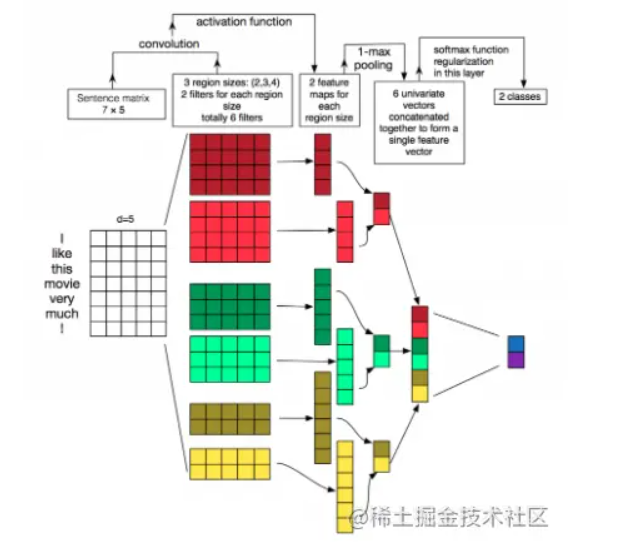1. Convert words into vectors with word embedding
2. Connect word vectors to the matrix in batches
3. Put the word matrix into a layer similar to the hidden layer of the neural network using filters instead of weights. Each filter will represent a word category, such as food, courtesy, etc. After this layer, each word matrix will score on each word category.
4. We choose the highest score from all layers for each word category.
5. Classify text with softmax function.

For example, in the sentence, I love this movie the score for positive emotions will be high since we have the word love. Even though this or movie may not be particularly positive, CNN can still recognize this sentence as a positive sentence.

1. Good Classification Performance: As described in this article, although the simple CNN static model has little fine-tuning of its parameters, it can perform well even compared to more complex deep learning models (including some RNN models).
2. Parallel processing becomes possible. This is a more agile and efficient model than RNN.

### Problem

Padding Required: Padding is required before the first word and after the last word or the model to accept all words.

## Summary

This article introduced the basis of traditional neural networks, recurrent neural networks, LSTM, and converters. We discussed their respective advantages and disadvantages to illustrate why an updated model should be developed.

0 0 0
Share on

# Related Products

• ##Machine Learning Platform For AI

An end-to-end platform that provides various machine learning algorithms to meet your data mining and analysis requirements.

• ##Accelerated Global Networking Solution for Distance Learning

Alibaba Cloud offers an accelerated global networking solution that makes distance learning just the same as in-class teaching.

• ##AI Acceleration Solution

Accelerate AI-driven business and AI model training and inference with Alibaba Cloud GPU technology